## Strange Attractors

Interactive 3D simulations

By Juan Carlos Ponce Campuzano, 15/August/2018

### Introduction

The term 'Strange Attractor' is used to describe an attractor (a region or shape to which points are 'pulled' as the result of a certain process) that displays sensitive dependence on initial conditions (that is, points which are initially close on the attractor become exponentially separated with time). The most famous strange attractor is undoubtedly the Lorenz attractor - a three dimensional object whose body plan resembles a butterfly or a mask. The Lorenz attractor, named for its discoverer Edward N. Lorenz, arose from a mathematical model of the atmosphere .

Imagine a rectangular slice of air heated from below and cooled from above by edges kept at constant temperatures. This is our atmosphere in its simplest description. The bottom is heated by the earth and the top is cooled by the void of outer space. Within this slice, warm air rises and cool air sinks. The state of the atmosphere in this model can be described by three time-evolving variables

• x = the convective flow
• y = the horizontal temperature distribution
• z = the vertical temperature distribution
with three parameters describing the character of the model itself
• $\sigma$ [sigma] = the ratio of viscosity to thermal conductivity
• $\rho$ [rho] = the temperature difference between the top and bottom of the slice
• $\beta$ [beta] = the width to height ratio of the slice
and a system of three ordinary differential equations describing the appropriate laws of fluid dynamics $$\left\{ \begin{eqnarray*} \frac{dx}{dt}&=&\sigma (-x + y)\\ \frac{dy}{dt}&=& -x z + \rho x - y \\ \frac{dz}{dt}&=& x y - \beta z \end{eqnarray*}\right.$$ When $\rho$ = 28, $\sigma$ = 10, and $\beta$ = 8/3, a solution curve of this system has the shape of the iconic butterfly and the position of particles will follow a similar path.

The Lorenz attractor was the first strange attractor, but there are many systems of equations that give rise to strange attractors. In the next section you will find simulations of strange attractors with particles moving based on the given system of ordinary differential equations, including one (or two) solution curve(s). Change the parameters to observe the behaviour of the particles and the solution curve(s). To access the simulation just click on the image.

### Interactive simulations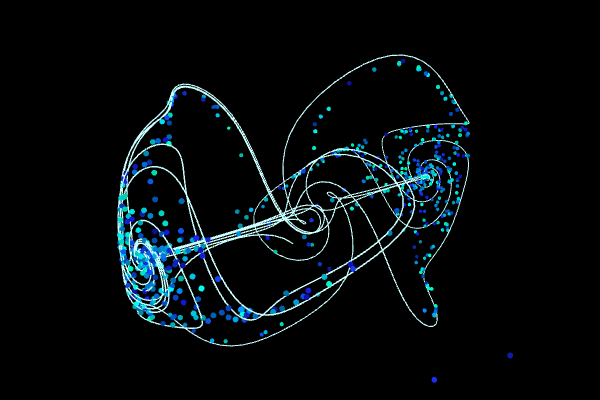### Thomas

Parameter:

$b=0.208186$

System:

$\left\{ \begin{eqnarray*} \frac{dx}{dt}&=&\sin y -bx\\ \frac{dy}{dt}&=&\sin z -by\\ \frac{dz}{dt}&=&\sin x-bz \end{eqnarray*}\right.$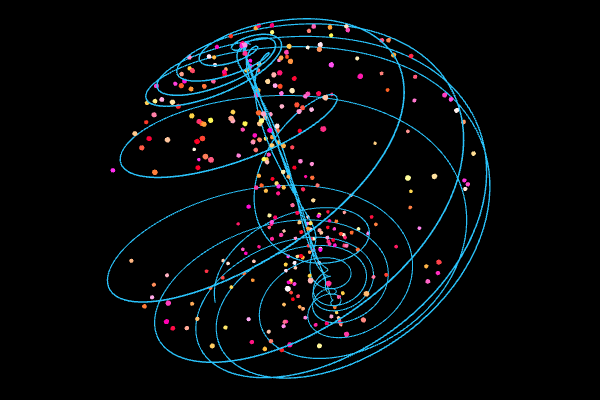### Aizawa

Parameters:

$a = 0.95,$ $b = 0.7,$ $c = 0.6,$
$d = 3.5,$ $e = 0.25,$ $f = 0.1$

System:

$\left\{ \begin{eqnarray*} \frac{dx}{dt}&=&(z - b) x - d y\\ \frac{dy}{dt}&=& d x + (z - b) y\\ \frac{dz}{dt}&=&c + a z - \frac{z^3}{3} -\\& &\;(x^2+y^2)(1+e z)+f z x^3 \end{eqnarray*}\right.$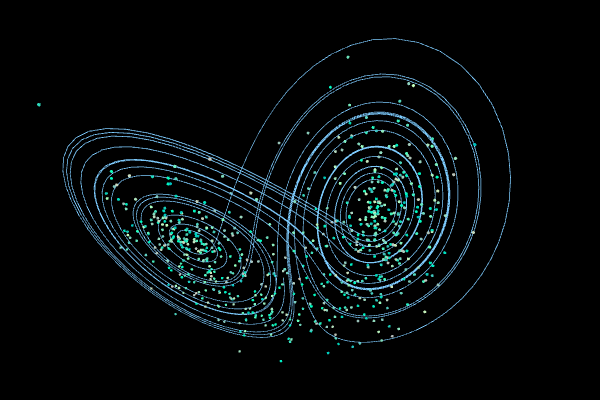### Lorenz

Parameters:

$\sigma = 10,$ $\rho = 28,$ $\beta = 8/3$

System:

$\left\{ \begin{eqnarray*} \frac{dx}{dt}&=&\sigma (-x + y)\\ \frac{dy}{dt}&=& -x z + \rho x - y \\ \frac{dz}{dt}&=& x y - \beta z \end{eqnarray*}\right.$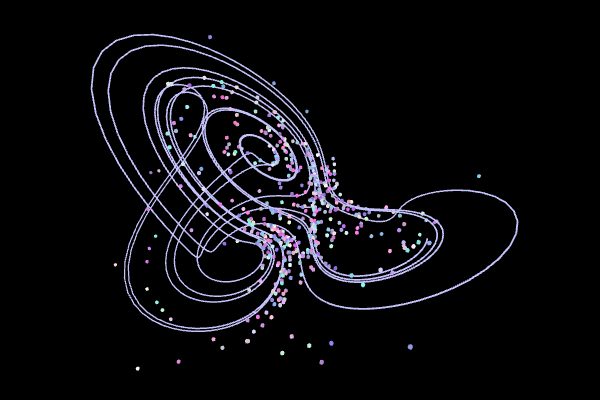Parameters:

$a = 3,$ $b = 2.7,$ $c = 1.7,$
$d = 2,$ $e = 9$

System:

$\left\{ \begin{eqnarray*} \frac{dx}{dt}&=& y - a x +b y z\\ \frac{dy}{dt}&=& c y - x z +z\\ \frac{dz}{dt}&=& d x y - e z \end{eqnarray*}\right.$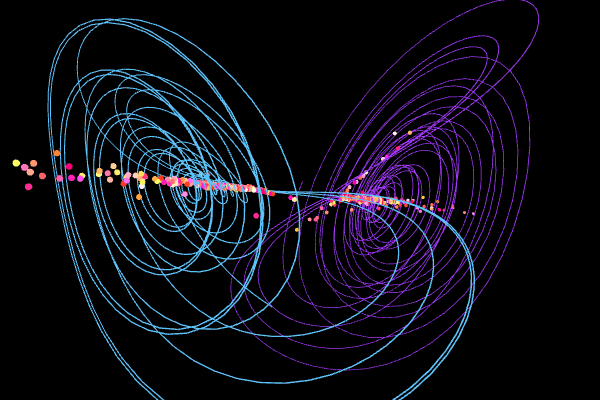### Chen

Parameters:

$\alpha = 5$, $\beta = -10$, $\delta = -0.38$

System:

$\left\{ \begin{eqnarray*} \frac{dx}{dt}&=&\alpha x- y z\\ \frac{dy}{dt}&=&\beta y + x z \\ \frac{dz}{dt}&=&\delta z + x y/3 \end{eqnarray*}\right.$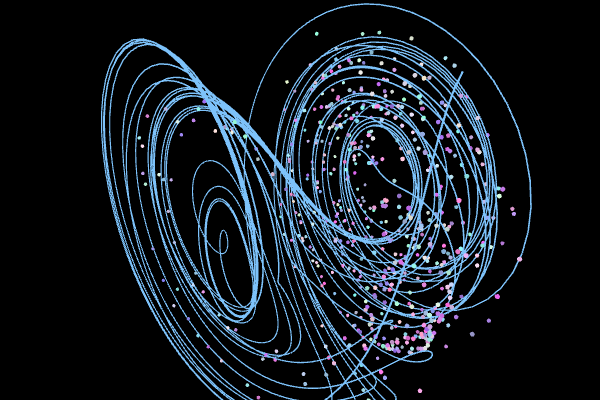### Lorenz83

Parameters:

$a = 0.95,$ $b = 7.91,$ $f = 4.83,$ $g = 4.66$

System:

$\left\{ \begin{eqnarray*} \frac{dx}{dt}&=&-a x - y^2 - z^2 + a f\\ \frac{dy}{dt}&=& -y + x y - b x z + g\\ \frac{dz}{dt}&=& -z + b x y + x z \end{eqnarray*}\right.$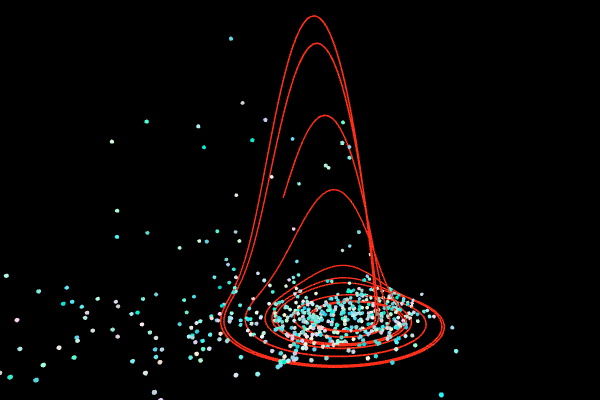### Rössler

Parameters:

$a = 0.2$, $b = 0.2$, $c = 5.7$

System:

$\left\{ \begin{eqnarray*} \frac{dx}{dt}&=&-(y+z)\\ \frac{dy}{dt}&=&x+ay\\ \frac{dz}{dt}&=&b+z(x-c) \end{eqnarray*}\right.$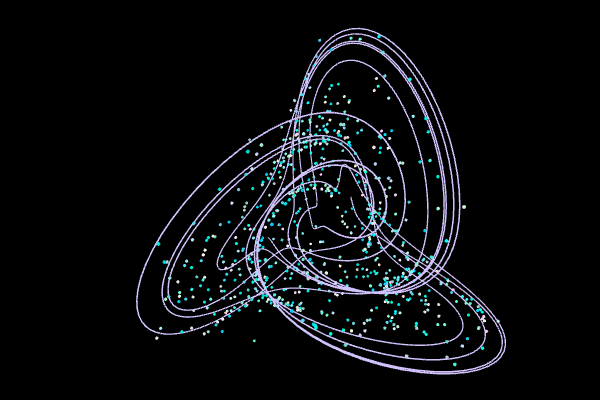### Halvorsen

Parameter:

$a = 1.89$

System:

$\left\{ \begin{eqnarray*} \frac{dx}{dt}&=&-a x-4y-4z-y^2 \\ \frac{dy}{dt}&=& -a y-4z-4x-z^2 \\ \frac{dz}{dt}&=& -a z-4x-4y-x^2 \end{eqnarray*}\right.$### Rabinovich-Fabrikant

Parameters:

$\alpha = 0.14$, $\gamma = 0.10$

System:

$\left\{ \begin{eqnarray*} \frac{dx}{dt}&=&y ( z - 1 + x^2 ) + \gamma x\\ \frac{dy}{dt}&=&x ( 3 z + 1 - x^2 ) + \gamma y\\ \frac{dz}{dt}&=& - 2 z ( \alpha + x y ) \end{eqnarray*}\right.$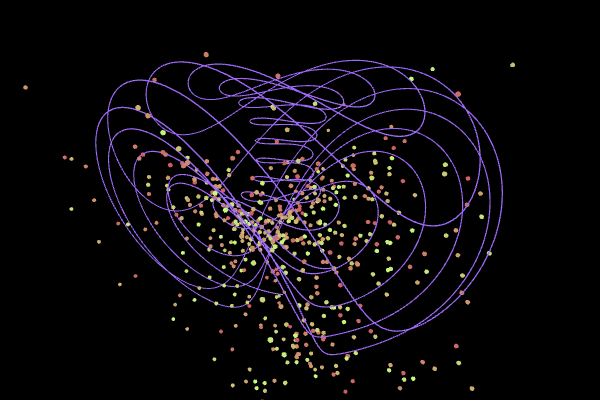### Three-Scroll Unified Chaotic System

Parameters:

$a = 32.48,$ $b = 45.84,$ $c = 1.18,$
$d = 0.13,$ $e = 0.57$, $f = 14.7$

System:

$\left\{ \begin{eqnarray*} \frac{dx}{dt}&=&a(y-x)+d x z \\ \frac{dy}{dt}&=& bx-x z + f y \\ \frac{dz}{dt}&=& cz+xy-ex^2 \end{eqnarray*}\right.$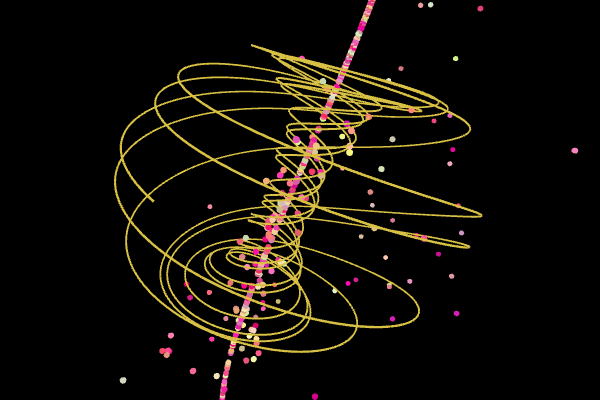### Sprott

Parameters:

$a = 2.07$, $b = 1.79$

System:

$\left\{ \begin{eqnarray*} \frac{dx}{dt}&=& y + a x y +x z \\ \frac{dy}{dt}&=& 1 - b x^2 +yz \\ \frac{dz}{dt}&=& x-x^2-y^2 \end{eqnarray*}\right.$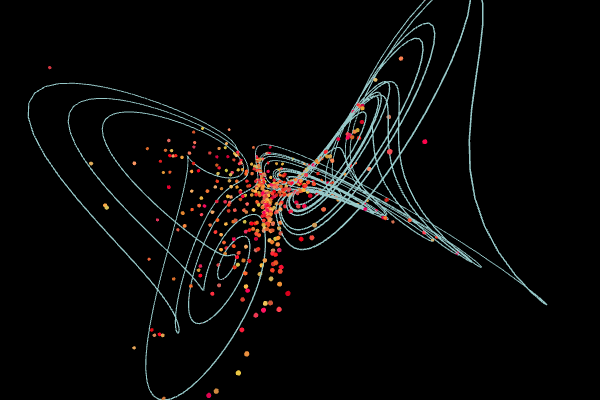### Four-Wing

Parameters:

$a = 0.2,$ $b = 0.01,$ $c = -0.4$

System

$\left\{ \begin{eqnarray*} \frac{dx}{dt}&=& ax+yz \\ \frac{dy}{dt}&=& b x + cy - xz \\ \frac{dz}{dt}&=& -z-xy \end{eqnarray*}\right.$

The souce code can be found here. For the 3d enviroment I used the p5.EasyCam library developed by Thomas Diewald. I used the dat.GUI library for the controls. The position of a particle is computed with a 4th order Runge-Kutta method.

Finally, if you found this site useful, you can support in Patreon or ❤️ PayPal or ❤️ Buy a T-shirt

I sincerely appreciate it. Thank you! 😃

1. Dadras, S., Momeni, H.R. (2009). A novel three-dimensional autonomous chaotic system generating two, three and four-scroll attractors. Physics Letters A. Volume 373, Issue 40. pp. 3637-3642.
2. Lorenz E. N. (1963). Deterministic Nonperiodic Flow. Journal of the Atmospheric Sciences. 20(2): 130–141.
3. Pan, L., Zhou, W., Fang,J., Li, D. (2010). A new three-scroll unified chaotic system coined. International Journal of Nonlinear Science. vol. 10, 462-474.
4. Rössler, O. E. (1976). An Equation for Continuous Chaos. Physics Letters, 57A (5): 397–398.
5. Solís Pérez, J. E., Gómez-Aguilar, J. F., Baleanu, D., Tchier, F. (2018). Chaotic Attractors with Fractional Conformable Derivatives in the Liouville–Caputo Sense and Its Dynamical Behaviors. Entropy. 2018, 20(5), 384.
6. Sprott. J. C. (2014). A dynamical system with a strange attractor and invariant tori Physic Letters A, 378 1361-1363.
7. Thomas, René. (1999). Deterministic chaos seen in terms of feedback circuits: Analysis, synthesis, ‘labyrinth chaos’. Int. J. Bifurcation and Chaos. 9 (10): 1889–1905.
8. Tam L., Chen J., Chen H., Tou W. (2008). Generation of hyperchaos from the Chen–Lee system via sinusoidal perturbation. Chaos, Solitons and Fractals. Vol. 38, 826-839.
9. Wang, Z., Sun, Y., van Wyk, J. B, Qi, G, van Wyk, M. A. (2009). A 3-D four-wing attractor and its analysis. Brazilian Journal of Physics, vol. 39, no. 3. pp.547-553.